# Python基础入门编程代码练习（一）

## 一、求两数之和

s1=int(input('请输入第1个数字：'))
s2=int(input('请输入第2个数字：'))
s3=s1+s2
print('这两数之和是：'+ str(s3))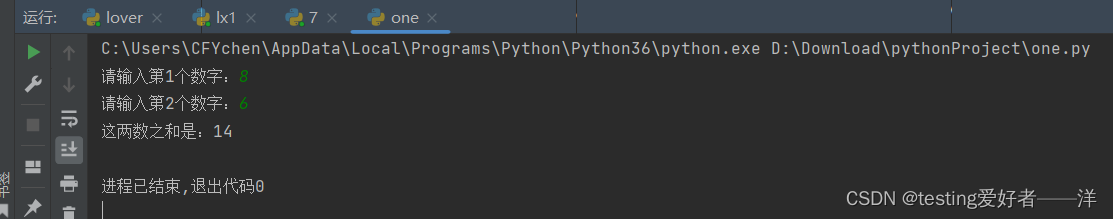## 二、输出商品价目表

print(  '\t' '\t'  '购物清单' '\n' '商品名称' '\t' '购买数量' '\t'
'商品单价' '\t' '金额' '\n' '鳄鱼夹克' '\t' '\t' '1' '\t'
'1900' '\t' '1900' '\n' 'ipodMP4' '\t' '\t' '2' '\t'
'400' '\t' '\t' '800')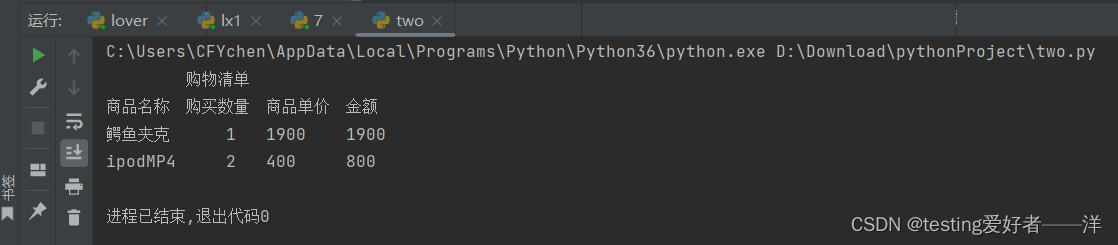## 三、计算周数和剩余天数

a=input('请输入天数:')
b =int(a)
c =b/7
d =b%7
print('周数是：%d\n剩余天数是：%d' %(c,d))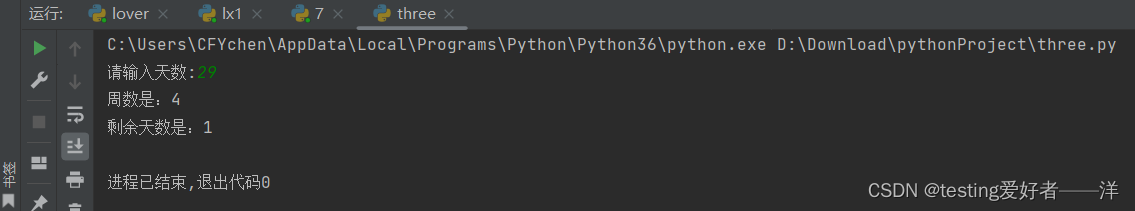## 四、已知圆的半径，求圆的面积及周长

class Yuan():
def __init__(self,r,area,C):
self.r = r
self.area = area
self.C = C

r=int(input('请输入此圆半径长度：'))
area=3.14*r*r
C=2*3.14*r

print("此圆半径为 %s 厘米 ,此圆的面积为 %s平方厘米；此圆周长为 %s厘米" % (r, area,C))

YUAN1 = Yuan("r","3.14*r","2*3.14*r")## 五、猜拳游戏

猜拳游戏

import random
choice=['剪刀','石头','布']
keepgoing=input('你想出剪刀,石头,布还是(Q)？')
while keepgoing!='Q':
computer=random.choice(choice)
print('你选择的是' +keepgoing+ '计算机选择的是' +computer)

if keepgoing==computer:
print('打平了')
elif keepgoing=='剪刀':
if computer=='石头':
print('计算机赢了')
else:
print('你赢了')
elif keepgoing=='石头':
if computer=='布':
print('计算机赢了')
else:
print('你赢了')
elif keepgoing=='布':
if computer=='剪刀':
print('计算机赢了')
else:
print('你赢了')
else:
print('你的结果不在范围类！！！')
print()
keepgoing=input('你想出剪刀,石头,布还是(Q)？')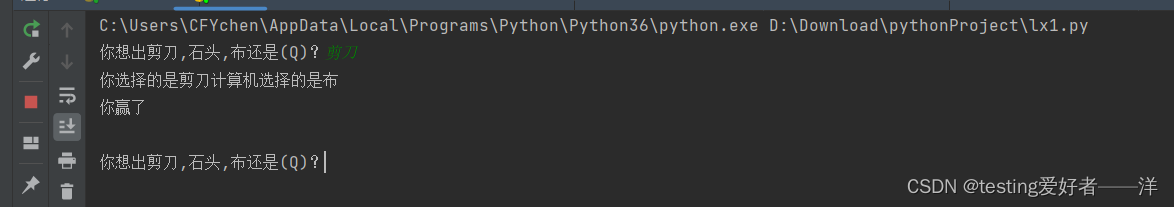## 六、买彩票

1. 如果体彩中了500万，我买车、资助希望工程、去欧洲旅游
2. 如果没中，我买下一期体彩，继续烧高香

text1=input('这一期是否中了体彩?')
if text1=='是':
print('买车、资助希望工程、去欧洲旅游')
else:
print('买下一期，继续烧高香')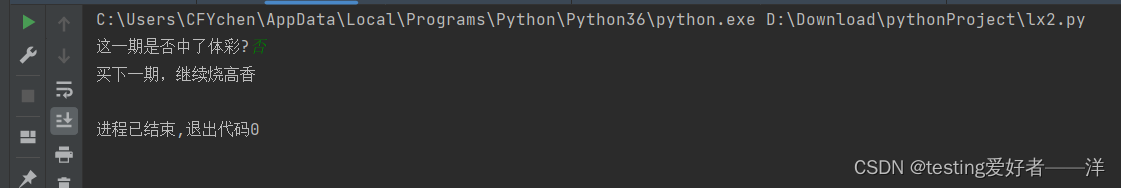## 七、实现幸运抽奖

1. 抽奖规则：会员号的百位数字等于产生的随机数字即为幸运会员

import random
text1 =input('我行我素购物管理系统 》 幸运抽奖')
random1 =random.randint(0,9)
#print(random1)
text2 =input('请输入4位会员号：')
a =int(text2)
b =int(a/100%10)
#print(b)
if b==random1:

print(text2 + '客户是幸运客户，获得精美Mp3一个。')
else:

print(text2+'号客户，谢谢您的支持！' )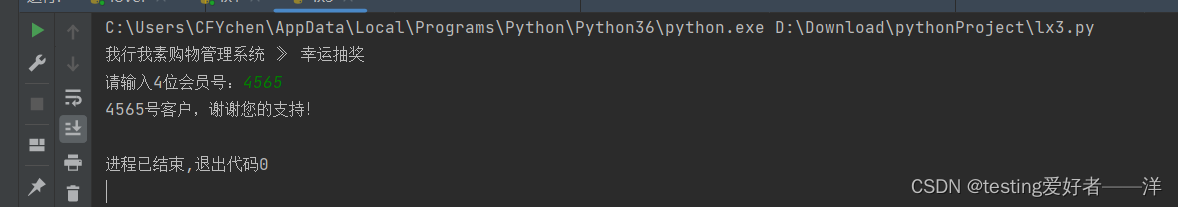## 八、产生随机数

1. 实现思路
1. 产生随机数
2. 从控制台接收一个4位会员号
3. 分解获得百位数
4. 判断是否是幸运会员
2. 产生随机数（0~9）的方法

random.randint(0,9)

import random
print('浩浩抽奖 > 幸运抽奖\n')
num = input('请输入4位会员号\t')
NewNum = int(num)
a = NewNum/100%10
rd = 2
rd = random.randint(0,9)
if a == rd:
print('幸运数字是%d 恭喜%d号客户是幸运观众，获得电视机一台' % (rd, NewNum))
else:
print('幸运数字是%d %d号客户，谢谢您的参与!' % (rd, NewNum))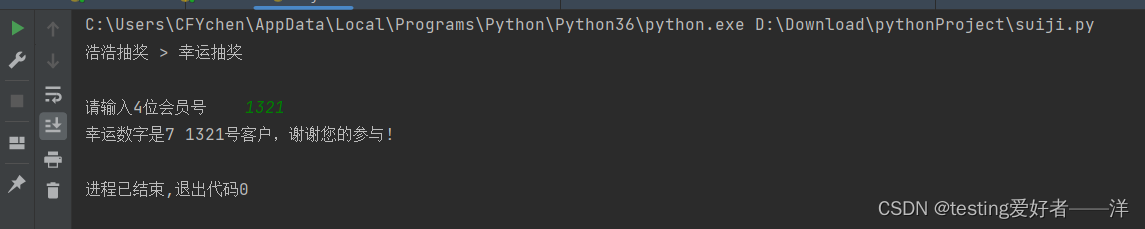## 九、会员信息录入

1. 录入会员信息
2. 判断录入的会员号是否合法

实现代码如下：

text1 =input('我行我素购物管理系统 》 客户信息管理 》 添加客户信息')
text2 =input('请输入会员号（<4位整数>)：')
a = int(text2)
if 1000 <= a <= 9999:
a = int(text2)
text3 = input('请输入会员生日（月/日<用两位数表示>）：')
text4 = input('请输入积分：')
print("已录入的会员信息是：" + text2, text3, text4)
else:
print('请重新输入会员号（<4位整数>)：')

运行结果：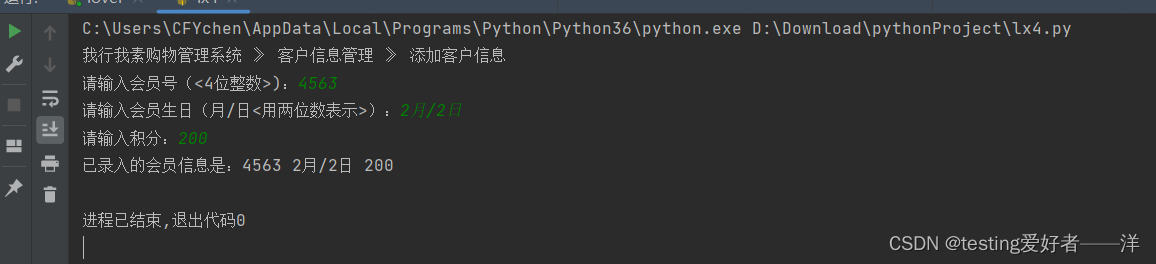## 十、考试成绩

1. 输入小明的考试成绩，显示所获奖励
1. 成绩==100分，爸爸给他买辆车
2. 100>成绩>=90分，妈妈给他买MP4
3. 90>成绩>=60分，妈妈给他买本参考书

实现代码如下：

text1 =int(input('小明的考试成绩：'))
if text1 ==100:
print('爸爸给你买辆车！')
elif 90<=text1<100:
print('妈妈给他买MP4！')
elif 60<=text1<90:
print('妈妈给他买本参考书！')
else:
print('什么都不给你买！等着被打！')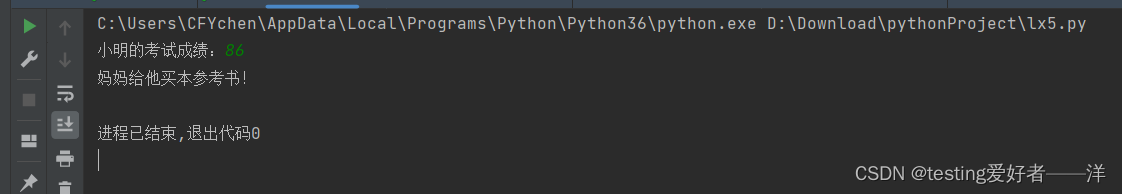## 十一、购物结算

嵌套if选择结构

1. 实现思路
1. 外层判断是否是会员
2. 内层判断是否达到相应打折要求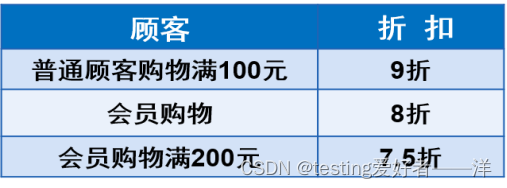实现代码如下：

test1 =str(input('请输入是否是会员：是（y）/否（其他字符）'))
test2 = int(input('请输入购物金额：'))

if test1 =='y':

if test2 >= 200:

print("实际支付：", test2*0.75)
else:

print("实际支付：", test2*0.8)
else:

if test2 >= 100:

print("实际支付：", test2*0.9)
else:
print("实际支付：", test2)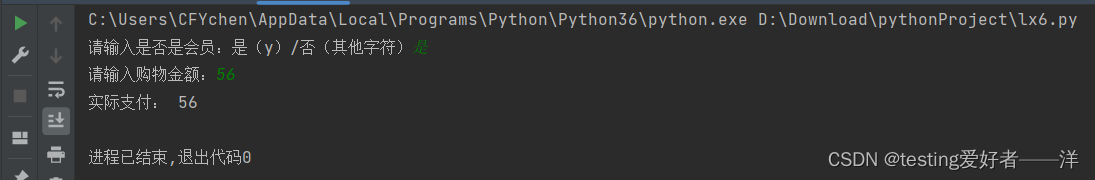被折叠的  条评论 为什么被折叠?到【灌水乐园】发言AE—Darren洋

¥1 ¥2 ¥4 ¥6 ¥10 ¥20获取中扫码支付点击重新获取扫码支付1.余额是钱包充值的虚拟货币，按照1:1的比例进行支付金额的抵扣。
2.余额无法直接购买下载，可以购买VIP、付费专栏及课程。余额充值